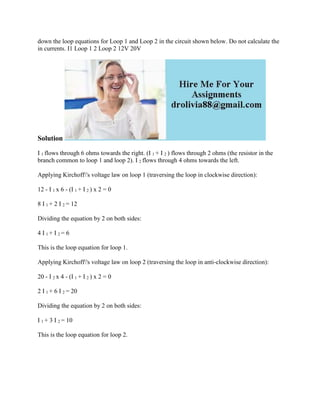Diese Präsentation wurde erfolgreich gemeldet.

# down the loop equations for Loop 1 and Loop 2 in the circuit shown bel.docx

Anzeige
Anzeige
Anzeige
Anzeige
Anzeige
Anzeige
Anzeige
Anzeige
Anzeige
Anzeige
Anzeige×

1 von 2 Anzeige

# down the loop equations for Loop 1 and Loop 2 in the circuit shown bel.docx

down the loop equations for Loop 1 and Loop 2 in the circuit shown below. Do not calculate the in currents. I1 Loop 1 2 Loop 2 12V 20V
Solution
I 1 flows through 6 ohms towards the right. (I 1 + I 2 ) flows through 2 ohms (the resistor in the branch common to loop 1 and loop 2). I 2 flows through 4 ohms towards the left.
Applying Kirchoff\'s voltage law on loop 1 (traversing the loop in clockwise direction):
12 - I 1 x 6 - (I 1 + I 2 ) x 2 = 0
8 I 1 + 2 I 2 = 12
Dividing the equation by 2 on both sides:
4 I 1 + I 2 = 6
This is the loop equation for loop 1.
Applying Kirchoff\'s voltage law on loop 2 (traversing the loop in anti-clockwise direction):
20 - I 2 x 4 - (I 1 + I 2 ) x 2 = 0
2 I 1 + 6 I 2 = 20
Dividing the equation by 2 on both sides:
I 1 + 3 I 2 = 10
This is the loop equation for loop 2.
.

down the loop equations for Loop 1 and Loop 2 in the circuit shown below. Do not calculate the in currents. I1 Loop 1 2 Loop 2 12V 20V
Solution
I 1 flows through 6 ohms towards the right. (I 1 + I 2 ) flows through 2 ohms (the resistor in the branch common to loop 1 and loop 2). I 2 flows through 4 ohms towards the left.
Applying Kirchoff\'s voltage law on loop 1 (traversing the loop in clockwise direction):
12 - I 1 x 6 - (I 1 + I 2 ) x 2 = 0
8 I 1 + 2 I 2 = 12
Dividing the equation by 2 on both sides:
4 I 1 + I 2 = 6
This is the loop equation for loop 1.
Applying Kirchoff\'s voltage law on loop 2 (traversing the loop in anti-clockwise direction):
20 - I 2 x 4 - (I 1 + I 2 ) x 2 = 0
2 I 1 + 6 I 2 = 20
Dividing the equation by 2 on both sides:
I 1 + 3 I 2 = 10
This is the loop equation for loop 2.
.

Anzeige
Anzeige

### down the loop equations for Loop 1 and Loop 2 in the circuit shown bel.docx

1. 1. down the loop equations for Loop 1 and Loop 2 in the circuit shown below. Do not calculate the in currents. I1 Loop 1 2 Loop 2 12V 20V Solution I 1 flows through 6 ohms towards the right. (I 1 + I 2 ) flows through 2 ohms (the resistor in the branch common to loop 1 and loop 2). I 2 flows through 4 ohms towards the left. Applying Kirchoff's voltage law on loop 1 (traversing the loop in clockwise direction): 12 - I 1 x 6 - (I 1 + I 2 ) x 2 = 0 8 I 1 + 2 I 2 = 12 Dividing the equation by 2 on both sides: 4 I 1 + I 2 = 6 This is the loop equation for loop 1. Applying Kirchoff's voltage law on loop 2 (traversing the loop in anti-clockwise direction): 20 - I 2 x 4 - (I 1 + I 2 ) x 2 = 0 2 I 1 + 6 I 2 = 20 Dividing the equation by 2 on both sides: I 1 + 3 I 2 = 10 This is the loop equation for loop 2.